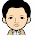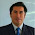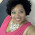Monday, 14 October 2013

Shapiro-Wilk Test Calculator

Either enter numbers as displayed below (must be three or more samples), or press choose file button to enter a single column CSV file (note: if you clear the textarea after loading a file, please reload page to be able to load the same file again..):

When you press calculate, the numbers entered above are sorted in ascending order of magnitude. A histogram of the data will be plotted as well.

Note that the results will be accurate for number of samples that lie between 3 and 5000 inclusive.

Result:
 Number of samples: Mean: Standard Deviation: Variance: Kurtosis: Calculated Shapiro-Wilk statistic W: Calculated Shapiro-Wilk p-value: Critical value of W (5% significance level):

Accept or reject Null Hypothesis..

 Histogram Frequency Values

For a given value of the Shapiro-Wilk statistic $W$, and the number of samples $N$, the p-value can be calculated as below. Simply enter the two values, and press the button below.

Enter $W$ value:-

Enter $N$ (number of samples):-

Calculated p-value..

1.2.3.4.5.